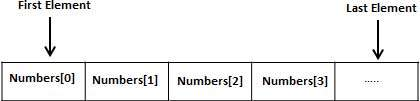# C# - Arrays

An array stores a fixed-size sequential collection of elements of the same type. An array is used to store a collection of data, but it is often more useful to think of an array as a collection of variables of the same type stored at contiguous memory locations.

Instead of declaring individual variables, such as number0, number1, ..., and number99, you declare one array variable such as numbers and use numbers, numbers, and ..., numbers to represent individual variables. A specific element in an array is accessed by an index.

All arrays consist of contiguous memory locations. The lowest address corresponds to the first element and the highest address to the last element.## Declaring Arrays

To declare an array in C#, you can use the following syntax −

```datatype[] arrayName;
```

where,

• datatype is used to specify the type of elements in the array.

• [ ] specifies the rank of the array. The rank specifies the size of the array.

• arrayName specifies the name of the array.

For example,

```double[] balance;
```

## Initializing an Array

Declaring an array does not initialize the array in the memory. When the array variable is initialized, you can assign values to the array.

Array is a reference type, so you need to use the new keyword to create an instance of the array. For example,

```double[] balance = new double;
```

## Assigning Values to an Array

You can assign values to individual array elements, by using the index number, like −

```double[] balance = new double;
balance = 4500.0;
```

You can assign values to the array at the time of declaration, as shown −

```double[] balance = { 2340.0, 4523.69, 3421.0};
```

You can also create and initialize an array, as shown −

```int [] marks = new int  { 99,  98, 92, 97, 95};
```

You may also omit the size of the array, as shown −

```int [] marks = new int[]  { 99,  98, 92, 97, 95};
```

You can copy an array variable into another target array variable. In such case, both the target and source point to the same memory location −

```int [] marks = new int[]  { 99,  98, 92, 97, 95};
int[] score = marks;
```

When you create an array, C# compiler implicitly initializes each array element to a default value depending on the array type. For example, for an int array all elements are initialized to 0.

## Accessing Array Elements

An element is accessed by indexing the array name. This is done by placing the index of the element within square brackets after the name of the array. For example,

```double salary = balance;
```

The following example, demonstrates the above-mentioned concepts declaration, assignment, and accessing arrays −

Live Demo

```using System;

namespace ArrayApplication {
class MyArray {
static void Main(string[] args) {
int []  n = new int; /* n is an array of 10 integers */
int i,j;

/* initialize elements of array n */
for ( i = 0; i < 10; i++ ) {
n[ i ] = i + 100;
}

/* output each array element's value */
for (j = 0; j < 10; j++ ) {
Console.WriteLine("Element[{0}] = {1}", j, n[j]);
}
}
}
}```

When the above code is compiled and executed, it produces the following result −

```Element = 100
Element = 101
Element = 102
Element = 103
Element = 104
Element = 105
Element = 106
Element = 107
Element = 108
Element = 109
```

## Using theforeachLoop

In the previous example, we used a for loop for accessing each array element. You can also use a foreach statement to iterate through an array.

Live Demo

```using System;

namespace ArrayApplication {
class MyArray {
static void Main(string[] args) {
int []  n = new int; /* n is an array of 10 integers */

/* initialize elements of array n */
for ( int i = 0; i < 10; i++ ) {
n[i] = i + 100;
}

/* output each array element's value */
foreach (int j in n ) {
int i = j-100;
Console.WriteLine("Element[{0}] = {1}", i, j);
}
}
}
}```

When the above code is compiled and executed, it produces the following result −

```Element = 100
Element = 101
Element = 102
Element = 103
Element = 104
Element = 105
Element = 106
Element = 107
Element = 108
Element = 109
```

## C# Arrays

There are following few important concepts related to array which should be clear to a C# programmer −

Sr.No. Concept & Description
1 Multi-dimensional arrays

C# supports multidimensional arrays. The simplest form of the multidimensional array is the two-dimensional array.

2 Jagged arrays

C# supports multidimensional arrays, which are arrays of arrays.

3 Passing arrays to functions

You can pass to the function a pointer to an array by specifying the array's name without an index.

4 Param arrays

This is used for passing unknown number of parameters to a function.

5 The Array Class

Defined in System namespace, it is the base class to all arrays, and provides various properties and methods for working with arrays.